# Online Civil Engineering Test - Previous UPSC Exam Papers Test 6Loading Test...

Instruction:

• This is a FREE online test. DO NOT pay money to anyone to attend this test.
• Total number of questions : 20.
• Time alloted : 30 minutes.
• Each question carry 1 mark, no negative marks.
• DO NOT refresh the page.
• All the best :-).

1.

What is the type of soil structure having arrangement of soil particles with a 'face to face' or parallel orientation generally recognized as

A.
 Honeycomb structureB.
 Single-grained structureC.
 Flocculent structureD.
 Dispersed structure2.

Given figure shows a retaining wall of base width B1 and height H1 The sp. gravity of the material of construction is S. Further AB = BC = CD =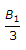When the depth of storage increases from 0 to H1, the resultant force will move from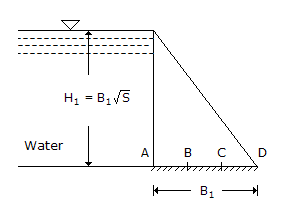A.
 A to BB.
 B to CC.
 C to DD.
 B to D3.

In each of the following examples certain quantity of work 'W' is done.
1. The point of application of a force of (50i + 60j + 80k) in kN units moves through a distance given by (6i + 5j + 2.5k) in metres.
2. A pump raises 2m3 of water through a height of 20 m (Take g = 10 m/s2).
3. A shaft delivering a torque = 200 kNm makes 0.75 revolution.
4. A couple (30i - 20j + 100k) in kNm units acting on a body rotates it through an angular displacement of (2i + 3j + 6k) radians.
The correct sequence of these quantities of work 'W' arranged in order of their increasing magnitude is

A.
 1, 4, 2, 3B.
 2, 4, 1, 3C.
 3, 2, 1, 4D.
 2, 3, 4, 14.

The fix of a plane table from three known points is good if

A.
 the middle station is the nearestB.
 the middle station is farther than the other two stationsC.
 either of the extreme stations is the nearestD.
 the middle station is close to the great circle5.

Consider the following statements regarding base-line measurements :
1. Very precise measurement can be done only on densely cloudy days or at nights.
2. Error due to application of non-standardised pull on the tape can be either cumulative or compensating type.
3. Intermediate obstructions in the measurement of base-lines can be cleared by erecting towers.
Of these statements :

A.
 1, 2 and 3 are correctB.
 1 and 2 are correctC.
 1 and 3 are correctD.
 2 and 3 are correct6.

The slenderness ratio of a compression member in the context of Rankine's formula is defined as

A.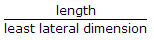B.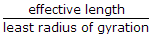C.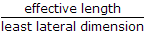D.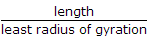7.

A VOR radio transmitter which emits beam in a vertical plane and gives an indication to the pilot whether he is to the left or right of the correct alignment for approach to the runway is known as

A.
 Outer markerB.
 Localiser antennaC.
 Glide slope antennaD.
 Marker beacon8.

When a single point load W travels over a simply supported beam, what is shape of the graph for maximum positive or negative shear force ?

A.
 A triangle with maximum ordinate W at centre and zero at two simple supportsB.
 A triangle with maximum ordinate W at a supportC.
 A rectangle with ordinate WD.
 A parabola with maximum ordinate W at centre of span and zero at supports9.

Which of the following pairs are correctly matched ?
1. Standard penetration test....Relative density
2. Vane shear....Cohesion
3. Consolidation test....Bearing capacity
Select the correct answer using the codes given below :

A.
 1, 2 and 3B.
 1 aloneC.
 1 and 2D.
 2 and 310.

Undrained strength of a clay soil can be obtained by conducting which of the following tests ?
1. UC test
2. Vane sheer test
3. Cyclic triaxial test
4. Slow direct sheer test
Select the correct answer using the code given below :

A.
 1 and 2 onlyB.
 2 onlyC.
 1 and 4D.
 1, 2 and 311.

The "Back sight" reading on a vertically held staff at a point A on the floor along the centre line of a railway tunnel is 3.465 m, and the "Fore sight" on the inverted staff held at the roof of the tunnel just vertically above A is 1.115 m. The height of the tunnel along the centre line at floor point A is

A.
 2.310 mB.
 3.465 mC.
 4.620 mD.
 6.930 m12.

As the state of strain of an element of dense sand changes from plain strain to trivial condition, the effective angle of internal friction :

A.
 increasesB.
 decreasesC.
 remains constantD.
 first increases and then remains constant13.

A concentrated load acting on the surface of a soil produces a stress of 19.1 kPa at a depth of 1 m below it. Using Boussinesq's expression, the stress at 2 m depth is

A.
 9.55 kPaB.
 6.37 kPaC.
 4.775 kPaD.
 4.37 kPa14.

For the purpose of designing a well point system for lowering ground water table in a sandy silt deposit, the coefficient of permeability of the soil is to be determined. Which one of the following methods would be most suitable ?

A.
 Constant Head Permeameter TestB.
 Variable Head Permeameter TestC.
 Pumping out Test in the fieldD.
 Pumping in Test in the field15.

In which one of the following grades of a highway is an emergency escape ramp provided ?

A.
 1 in 200B.
 Zero gradeC.
 DowngradeD.
 Upgrade16.

A 30 cm diameter friction pile is embedded 10 m into a homogeneous consolidated deposit. Unit adhesion developed between clay and pile shaft is 4 t/m2 and adhesion factor is 0.7. The safe load for factor of safety 2.5 will be

A.
 21.50 tB.
 11.57 tC.
 10.55 tD.
 6.35 t17.

In a cohesionless soil deposit having a unit weight of 1.5 t/m3and an angle of internal friction of 30° the active and passivelateral earth pressure intensities (in t/m2) at a depth of 10 m will, respectively, be

A.
 15 andB.
 5 and 15C.
 10 and 20D.
 20 and 1018.

Consider the following statements: An aqueduct is a cross drainage work in which
(a) a canal is carried over the drainage channel
(b) a drainage channel is carried over the canal
(c) both drainage channel and canal are at the same level.
Which of the statements given above is/are correct ?

A.
 1 onlyB.
 1 and 2 onlyC.
 2 and 3 onlyD.
 1, 2 and 319.

The distribution system configuration shown in the following figure is called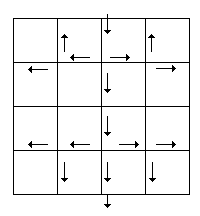A.
 dead end systemB.
 grid iron systemC.
 radial systemD.
 ring system20.

Permissible limit of cant deficiency for Broad Gauge (B.G.) is

A.
 50 mmB.
 60 mmC.
 75 mmD.
 88 mm